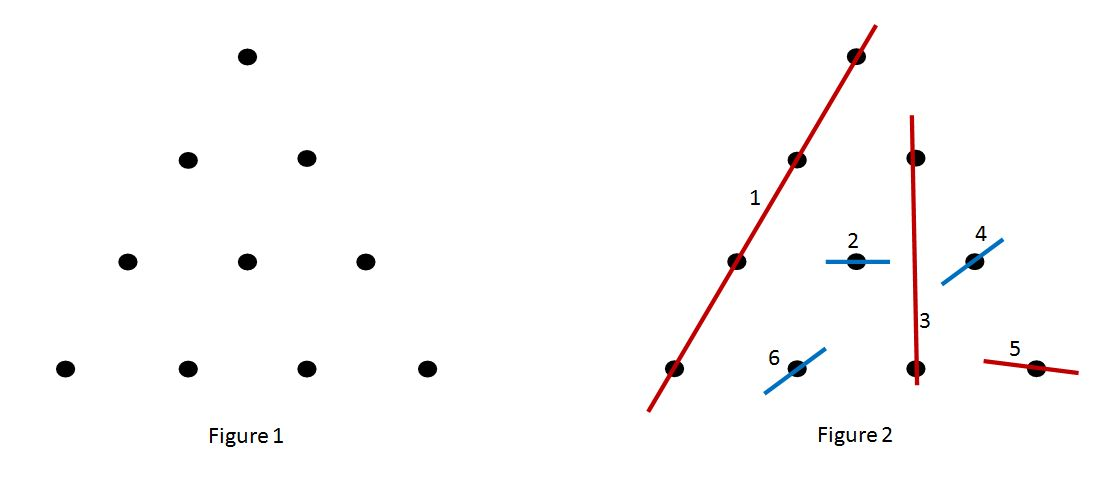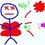# A game of triangles and linesThere is a game between two players that I have played and it is as follows:

Arrange $\frac{n(n+1)}{2}$ points in an equilateral triangle, such as in figure 1, when $n$ is 4. The players connect points with a single line segment at each turn, and each line segment must pass through at least one point. No two line segments can intersect each other. A player loses when there are no more possible lines to be drawn, like in figure 2. Red is the first player while Blue is the second. Blue has drawn the 6th line and there are no more lines for Red to draw, so Red loses.

Is there a winning strategy for any of the two players for a particular $n$?

To clarify, the line segments should be drawn as short as possible. Therefore, line 2 in figure 2 cannot be extended to prevent Red from drawing line 3.Note by Yu Tse Lee
7 years ago

This discussion board is a place to discuss our Daily Challenges and the math and science related to those challenges. Explanations are more than just a solution — they should explain the steps and thinking strategies that you used to obtain the solution. Comments should further the discussion of math and science.

When posting on Brilliant:

• Use the emojis to react to an explanation, whether you're congratulating a job well done , or just really confused .
• Ask specific questions about the challenge or the steps in somebody's explanation. Well-posed questions can add a lot to the discussion, but posting "I don't understand!" doesn't help anyone.
• Try to contribute something new to the discussion, whether it is an extension, generalization or other idea related to the challenge.

MarkdownAppears as
*italics* or _italics_ italics
**bold** or __bold__ bold
- bulleted- list
• bulleted
• list
1. numbered2. list
1. numbered
2. list
Note: you must add a full line of space before and after lists for them to show up correctly
paragraph 1paragraph 2

paragraph 1

paragraph 2

[example link](https://brilliant.org)example link
> This is a quote
This is a quote
    # I indented these lines
# 4 spaces, and now they show
# up as a code block.

print "hello world"
# I indented these lines
# 4 spaces, and now they show
# up as a code block.

print "hello world"
MathAppears as
Remember to wrap math in $$ ... $$ or $ ... $ to ensure proper formatting.
2 \times 3 $2 \times 3$
2^{34} $2^{34}$
a_{i-1} $a_{i-1}$
\frac{2}{3} $\frac{2}{3}$
\sqrt{2} $\sqrt{2}$
\sum_{i=1}^3 $\sum_{i=1}^3$
\sin \theta $\sin \theta$
\boxed{123} $\boxed{123}$

Sort by:

If people like this game, they should check out this variation from Yong See F.

Staff - 7 years ago

This game appears to be winning for player 2 for n=4. Player 2 must follow symmetry with player 1 as long as possible. This ensures that always an even number of points are left to be 'lined' which results in player 2 winning.

If player 1 starts with line 3 from the example the symmetry-strategy goes wrong though...

- 7 years ago

Well the symmetry strategy does go wrong but then I can use the method for $n = 2k - 1$ which you yourself have shown in the post below :)

I believe that n=4 is winning for player 2, but can only think of brute force as a way to prove that it is true.

- 7 years ago

I don't get it, you say that the line should be as short as possible but in your figure 2, line no. 1 contradicts that.

No, basically if one wants to connect one point, he cannot extend the line so far that it almost touches the second point; if he wants to connect four points, it is allowed to connect all four, as long as the line does not extend past that.

- 7 years ago

We start with $n=2$. This position is obviously losing.

For $n=2k-1$ $(k=1,2,3,...)$ we draw a line through the top and the middle point at the bottom and proceed to win by mirroring our opponents moves in that line.

That just leaves us $n=2k$ $(k=2,3,4,...)$ to consider. At this point I don't see a strategy to win, so perhaps this always loses?

- 7 years ago

I see what you are doing for $n=2k-1$, but that only works if you go first. $n=2k$ seems pretty interesting!

- 7 years ago

"but that only works if you go first"

Ah, I assumed the opponent plays optimally, but that doesn't have to be the case of course. It makes it nearly impossible to come up with strategies though, since the number of possible opening moves increases dramatically with $n$.

- 7 years ago